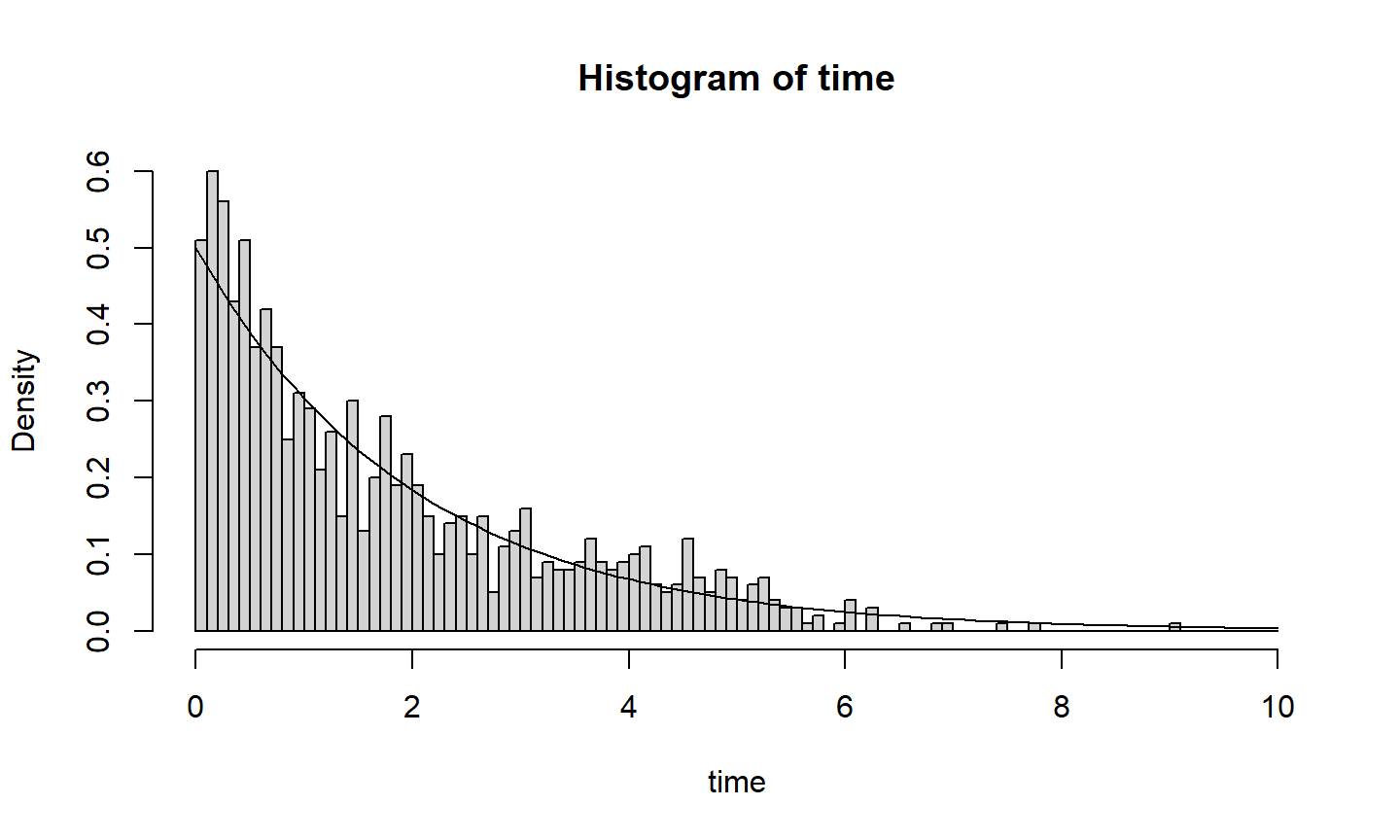## What are queues

• Items (customers, patients, cars) enter a system (check-out, hospital, repair shop) for service.

• They may have to wait in-line, in the queue, to be serviced.

• When they finally receive service, this also takes time.

• When they are done, they depart the system, this may also take time.

• Queuing time and service time, (and departure time) are often considered together.

• We are interested in quantities such as how many items are in a system at a time $$t$$, average time in the system, and the stability of the queue.

## Some terminology and common assumptions

• The discipline of a queue refers to how items are serviced: first in first out (FIFO) being a common assumption.

• Usually one or more servers.

• Arrival time to the queue are often assumed to correspond to a renewal process.

• Service times are often assumed to be i.i.d.

• Technical maths tools that are used in analysing queues include finding hidden Markov chains.

## Kendall notation

• A/S/c; three parameters denoting the inter-arrival times, service times, and the number of servers.

• Inter-arrival times and service times are i.i.d. and the queue is by default FIFO.

• M stands for Markov (exponential times)

• M/M/1 or $$M(\lambda)/M(\mu)/1$$ refers to a queue with one server, where inter-arrival and service times are exponential (with not necessary the same rate).

• G/G/1 refers to a general (and not necessarily the same) distribution for arrivals and service.

## Code M/M/1

Hidden in an early exercise, we had considered such a queue, with $$\lambda = 1$$ and $$\mu = 1.5$$. We had code that produced a histogram of the total an item spends in such a system.

inter=rexp(1000, 1)
arr=cumsum(inter)
service = rexp(1000,1.5)

output <- arr + service
for (i in 1:999){
if (arr[i+1] < output[i]){output <- c(output, output[i] + service[i+1])}
if (arr[i+1] > output[i]){output <- c(output, arr[i+1] + service[i+1])}
output
}

## continued

time = output - arr
x = seq(0,max(time)+1, by=0.01)
b = seq(0,max(time)+1, by=0.1)
hist(time, prob=T, breaks=b)
curve(dexp(x,0.5), add=T)## General results

The ratio of the expected service time over expected inter-arrival time is the traffic intensity. Let $$Q(t)$$ be the number of customers in the system (waiting and being serviced) at time $$t$$. We say that $$Q$$ is stable if the law of $$Q(t)$$ converges to a probability distribution as $$t \to \infty$$. For a G/G/1 queue, we have:

• If the traffic intensity is strictly less than $$1$$, then $$Q$$ is stable

• If the traffic intensity is greater than $$1$$, then $$Q$$ is not stable

• If the traffic intensity is $$1$$, then $$Q$$ is not stable, provided one of the service time or inter-arrival time is not deterministic.

## Little’s law; $$L = \lambda W$$;

Exact version in $$[0,T]$$: For a system that is empty at $$0$$ and $$T$$

• $$Q(t)$$ = number of items in the system at time $$t$$

• $$\lambda$$ = average arrival rate in $$[0,T]$$

• $$N$$ = the total number of items arriving in $$[0,T]$$

• $$L$$ = average number of items in the system in $$[0,T]$$

• $$W$$ =average waiting time of an item in $$[0,T]$$

• $$A = \int_0^T Q(t)dt$$ area under $$Q$$ curve

## Proof

Here average is defined so that

• $$L = A/T$$

• $$\lambda = N/T$$

• $$W = A/N$$

• Hence $L = (A/T) = (A/N) * (N/T) = \lambda W.$